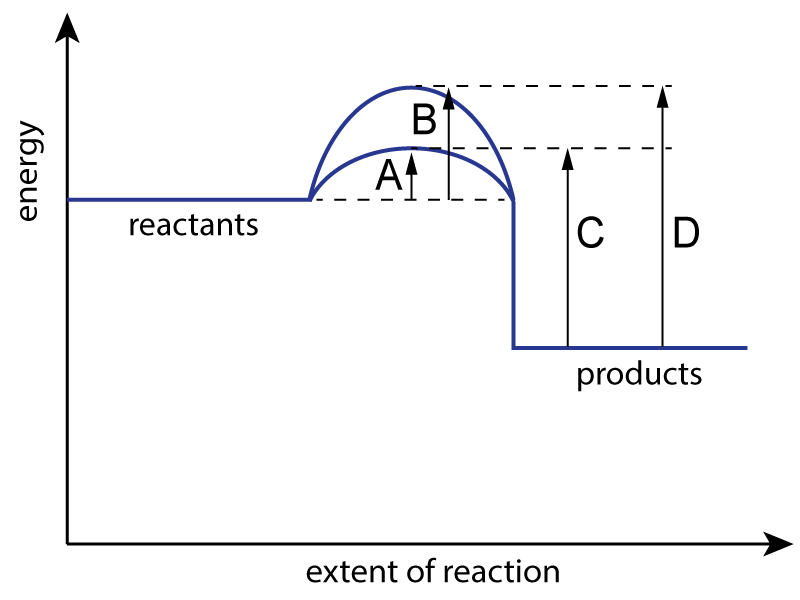Triple science: iGCSE Chemistry Only

Q1-2:
The energy level diagram for a reaction with and without a catalyst is shown below:1. Which letter represents the activation energy for the uncatalysed reaction?
2. Which letter represents the activation energy for the catalyzed reaction?

Q3-4:

An energy level diagram for a reaction is shown here:3. Which type of energy change does this diagram represent and what sign should ΔH be given?

 Type of change Sign of ΔH A exothermic positive B exothermic negative C endothermic positive D endothermic negative

4.For which reaction(s) would this be an appropriate diagram?

 I. CH4 + 2O2 → CO2 + 2H2O II. C + O2 →  CO2 III. 2H2+ O2 → 2H2O

• A.   all of them
• B.   I and II only
• C.  II and III only
• D.   none of them

5. Which of the following statements about catalysts are true? A catalyst..

 I. provides an alternative pathway with lower activation energy II. provides an alternative pathway with a lower enthalpy change III. is chemically unchanged at the end of the reaction

• A.   I and II
• B.   I and III
• C.   II and III
• D.   all of them

6. Which of the following is correct about the energy changes involved during bond breaking and bond making?

 Bond breaking Bond making A exothermic endothermic B exothermic exothermic C endothermic endothermic D endothermic exothermic

7. For the reaction:

R2 + S2  2RS

Which combination of bond strengths would produce the most exothermic reaction?

 Bond strength in R2 and S2 Bond strength in RS A weak weak B weak strong C strong weak D strong strong

Q8-10:
The following equation represents the conversion of sulfur dioxide to sulfur trioxide:Average bond energies for the bonds involved are:
O=O bond          496 kJ/mol
S=O bond           523 kJ/mol

8. The energy required to break the bonds in the reactants is..
• A.   1542 kJ/mol
• B.   1569 kJ/mol
• C.   2588 kJ/mol
• D.   3138 kJ/mol
9. The energy given out when the bonds in the products form is..
• A.   1542 kJ/mol
• B.   1569 kJ/mol
• C.   2588 kJ/mol
• D.   3138 kJ/mol
10. The enthalpy change, ΔH, for this reaction is..
• A.   +550 kJ/mol
• B.   -550 kJ/mol
• C.   +27 kJ/mol
• D.  -27 kJ/mol